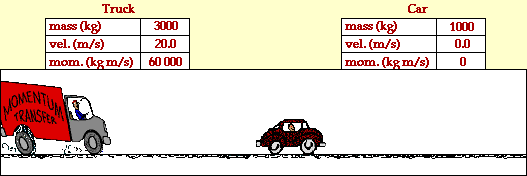## Truck Rear Ends Car

### Elastic Collision

Collisions between objects are governed by laws of momentum and energy. When a collision occurs in an isolated system, the total momentum of the system of objects is conserved. Provided that there are no net external forces acting upon the objects, the momentum of all objects before the collision equals the momentum of all objects after the collision. If there are only two objects involved in the collision, then the momentum lost by one object equals the momentum gained by the other object.

Certain collisions are referred to as elastic collisions. Elastic collisions are collisions in which both momentum and kinetic energy are conserved. The total system kinetic energy before the collision equals the total system kinetic energy after the collision. If total kinetic energy is not conserved, then the collision is referred to as an inelastic collision.

The animation below portrays the elastic collision between a 3000-kg truck and a 1000-kg car. The before- and after-collision velocities and momentum are shown in the data tables.In the collision between the truck and the car, total system momentum is conserved. Before the collision, the momentum of the truck is 60000 kg*m/s and the momentum of the car is 0 kg*m/s; the total system momentum is 60000 kg*m/s. After the collision, the momentum of the truck is 30000 kg*m/s and the momentum of the car is 30000 kg*m/s; the total system momentum is 60000 kg*m/s. The total system momentum is conserved. The momentum lost by the truck (30000 kg*m/s) is gained by the car.

An analysis of the kinetic energy of the two objects reveals that the total system kinetic energy before the collision is 600000 Joules (600000 J for the truck plus 0 J for the car). After the collision, the total system kinetic energy is 600000 Joules (150000 J for the truck and 450000 J for the car). The total kinetic energy before the collision is equal to the total kinetic energy after the collision. A collision in which total system kinetic energy is conserved is known as an elastic collision.

For more information on physical descriptions of motion, visit The Physics Classroom Tutorial. Detailed information is available there on the following topics:

Momentum

Momentum Conservation Principle

Isolated Systems

Momentum Conservation in Collisions

Kinetic Energy